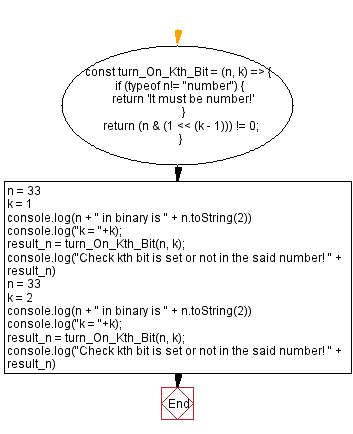# JavaScript - Check if kth bit is set or not for a number

## JavaScript Bit Manipulation: Exercise-11 with Solution

Write a JavaScript program to check whether the kth bit is set or not in a given number. Return true if the kth bit is set otherwise false.

In a binary representation the presence of a non-zero value indicates that the k'th bit is set.

Test Data:
(33, 1) -> true
Explanation:
Binary format of 33 is -> 100001
K =1 -> kth bit is 1 [Non-zero value indicates that the k'th bit is set.]
(33, 2) -> false
Explanation:
Binary format of 33 is -> 100001
K =2 -> kth bit is 0 [Zero value indicates that the k'th bit is not set.]

Sample Solution:

JavaScript Code:

``````const turn_On_Kth_Bit = (n, k) => {
if (typeof n!= "number") {
return 'It must be number!'
}
return (n & (1 << (k - 1))) != 0;
}
n = 33
k = 1
console.log(n + " in binary is " + n.toString(2))
console.log("k = "+k);
result_n = turn_On_Kth_Bit(n, k);
console.log("Check kth bit is set or not in the said number! " + result_n)
n = 33
k = 2
console.log(n + " in binary is " + n.toString(2))
console.log("k = "+k);
result_n = turn_On_Kth_Bit(n, k);
console.log("Check kth bit is set or not in the said number! " + result_n)
```
```

Sample Output:

```33 in binary is 100001
k = 1
Check kth bit is set or not in the said number! true
33 in binary is 100001
k = 2
Check kth bit is set or not in the said number! false
```

Flowchart:Live Demo:

See the Pen javascript-bit-manipulation-exercise-11 by w3resource (@w3resource) on CodePen.

* To run the code mouse over on Result panel and click on 'RERUN' button.*

Improve this sample solution and post your code through Disqus

What is the difficulty level of this exercise?

Test your Programming skills with w3resource's quiz.

﻿

## JavaScript: Tips of the Day

Returns the symmetric difference between two arrays, after applying the provided function to each array element of both

Example:

```const tips_symmetricDifference = (x, y, fn) => {
const sA = new Set(x.map(v => fn(v))),
sB = new Set(y.map(v => fn(v)));
return [...x.filter(x => !sB.has(fn(x))), ...y.filter(x => !sA.has(fn(x)))];
};

console.log(tips_symmetricDifference([3.5, 5.5], [5.5, 7.5], Math.floor));
```

Output:

```[3.5, 7.5]
```